# PHP Statistics Library using Al-Kashi PHP Statistical Analysis Functions - Al-Kashi package blog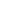All package blogs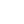Al-KashiAl-Kashi package blog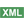PHP Statistics Librar...Post a comment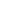See comments (13) Trackbacks (0)

Author:

Viewers: 153

Last month viewers: 57

Package: Al-Kashi

Al-Kashi is a project that aims to provide a rich PHP package full of useful statistical functions for online business intelligent and data mining.By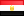ar-php.org

<email contact>

## Introduction to Al-Kashi PHP Statistics Class

The Al-Kashi is a project that can be used in applications that may incude an online log file analysis, advertising campaign statistics, or survey or voting results on-the-fly analysis.

This project is published under GPL license. You can download it from the PHP Classes web site. Here and you can see the log of changes .

## Example Data and Statistics

Below follows examples of statistics obtained with this class from example data set.

The data presented in this example was extracted from the 1974 Motor Trend US magazine. It comprises fuel consumption and 10 aspects of automobile design and performance for 32 cars 1973-74 models). You can download the example data file from here.

Description (Motor Trend Car Road Tests)

Format: A data frame with 32 observations on 12 variables.

 ID Title Description 1 model Car models 2 mpg Miles/(US) gallon 3 cyl Number of cylinders 4 disp Displacement (cu.in.) 5 hp Gross horsepower 6 drat Rear axle ratio 7 wt Weight (lb/1000) 8 qsec 1/4 mile time 9 vs V/S 10 am Transmission (0 = automatic, 1 = manual) 11 gear Number of forward gears 12 carb Number of carburetors

Example code read example data and feed it to Al Kashi

1. \$sep = "\t"\$nl  = "\n";
2.
3. \$content = file_get_contents('data.txt');
4.
5. \$records = explode(\$nl\$content);
8.
9. foreach (\$records as \$id=>\$record) {
10.     \$record = trim(\$record);
11.     if (\$record == ''continue;
12.
13.     \$fields = explode(\$sep\$record);
15.
16.     foreach (\$fields as \$field) {
17.         \$title = array_shift(\$titles);
18.         \$data[\$title][] = \$field;
19.     }
20. }
21.
22. \$x = \$data['wt'];
23. \$y = \$data['mpg'];
24.
25. require('kashi.php');
26.
27. \$kashi = new Kashi();

### PHP Statistical Functions Summary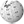Mean (x) 3.21725Mean (x, "geometric") 3.0701885671208Mean (x, "harmonic") 2.9182632148104Median (x) 3.325Mode (x) Array (  => 3.44 )Variance (x) 0.95737896774194SD (x) 0.9784574429897%CV (x) 30.412850819479Skewness (x) 0.46591610679299 Is it significant (i.e. test it against 0)? bool(false)Kurtosis (x) 0.41659466963493 Is it significant (i.e. test it against 0)? bool(false)Rank (x) 9, 12, 7, 16, 18, 21, 23, 15, 13, 18, 18, 29, 25, 26, 30, 32, 31, 6, 2, 3, 8, 22, 17, 27, 28, 4, 5, 1, 14, 10, 23, 11
1. // \$x is an array of values
2. echo 'Arithmetic Mean: ' . \$kashi->mean(\$x) . '<br>';
3. echo 'Aeometric Mean: '  . \$kashi->mean(\$x"geometric") . '<br>';
4. echo 'Harmonic Mean: '   . \$kashi->mean(\$x"harmonic")  . '<br>';
5.
6. echo 'Mode: '     . print_r(\$kashi->mode(\$x)) . '<br>';
7. echo 'Median: '   . \$kashi->median(\$x)   . '<br>';
8. echo 'Variance: ' . \$kashi->variance(\$x) . '<br>';
9. echo 'SD: '       . \$kashi->sd(\$x)       . '<br>';
10. echo '%CV: '      . \$kashi->cv(\$x)       . '<br>';
11.
12. echo 'Skewness: ' . \$kashi->skew(\$x) . '<br>';
13. echo 'Is it significant (i.e. test it against 0)? ';
14. var_dump(\$kashi->isSkew(\$x));
15.
16. echo 'Kurtosis: ' . \$kashi->kurt(\$x) . '<br>';
17. echo 'Is it significant (i.e. test it against 0)? ';
18. var_dump(\$kashi->isKurt(\$x));
19.
20. echo 'Rank (x): ';
21. echo implode(', '\$kashi->rank(\$x)) . '<br>';

### Statistical Graphics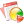Boxplot ```Array ( [min] => 1.513 [q1] => 2.62 [median] => 3.325 [q3] => 3.73 [max] => 5.282 [outliers] => Array (  => 5.345  => 5.424 ) ) ```Histogram ```Array ( [1.513-2.002] => 4 [2.002-2.491] => 4 [2.491-2.98] => 4 [2.98-3.469] => 9 [3.469-3.957] => 7 [3.957-4.446] => 1 [4.446-4.935] => 0 [4.935-5.424] => 3 ) ```Normal Q-Q Plot x = -0.62609901275838, -0.36012989155586, -0.83051087731871, -0.039176085543034, 0.27769043950814, 0.36012989155586, 0.62609901275838, -0.11776987461046, -0.27769043950814, 0.19709908415753, 0.11776987461046, 1.2298587580185, 0.72451438304624, 0.83051087731871, 1.417797139161, 2.1538746917937, 1.6759397215193, -0.94678175657479, -1.6759397215193, -1.417797139161, -0.72451438304624, 0.44509652516901, 0.039176085543034, 0.94678175657479, 1.0775155681381, -1.2298587580185, -1.0775155681381, -2.1538746917937, -0.19709908415753, -0.53340970683585, 0.53340970683585, -0.44509652516901y = 2.62, 2.875, 2.32, 3.215, 3.44, 3.46, 3.57, 3.19, 3.15, 3.44, 3.44, 4.07, 3.73, 3.78, 5.25, 5.424, 5.345, 2.2, 1.615, 1.835, 2.465, 3.52, 3.435, 3.84, 3.845, 1.935, 2.14, 1.513, 3.17, 2.77, 3.57, 2.78Ternary Plot x = 0.729, 0.722, 0.734, 0.706, 0.695, 0.675, 0.659, 0.723, 0.701, 0.692, 0.679, 0.663, 0.676, 0.654, 0.577, 0.574, 0.625, 0.779, 0.785, 0.788, 0.716, 0.667, 0.664, 0.645, 0.691, 0.763, 0.766, 0.796, 0.689, 0.723, 0.672, 0.718y = 0.356, 0.36, 0.369, 0.382, 0.376, 0.419, 0.407, 0.364, 0.406, 0.387, 0.408, 0.398, 0.395, 0.422, 0.463, 0.459, 0.403, 0.312, 0.317, 0.31, 0.394, 0.407, 0.417, 0.41, 0.368, 0.34, 0.323, 0.3, 0.375, 0.354, 0.381, 0.377
1. echo 'Boxplot: <br><pre>';
2. print_r(\$kashi->boxplot(\$x));
3. echo '</pre><br>';
4.
5. echo 'Histogram: <br><pre>';
6. print_r(\$kashi->hist(\$x, 8));
7. echo '</pre><br>';
8.
9. echo 'Normal Q-Q Plot: <br>';
10. \$qq = \$kashi->qqnorm(\$x);
11. echo 'x = ' . implode(', '\$qq['x']) . '<br>';
12. echo 'y = ' . implode(', '\$qq['y']) . '<br>';
13.
14. echo 'Ternary Plot: <br>';
15. \$xy = \$kashi->ternary(\$data['wt'], \$data['mpg'], \$data['qsec']);
16. echo 'x = ' . implode(', '\$xy['x']) . '<br>';
17. echo 'y = ' . implode(', '\$xy['y']) . '<br>';

### Correlation, Regression, and t-TestCovariance (x, y) -5.1166846774194Correlation (x, y) -0.86765937651723 Significant of Correlation 1.2939593840855E-10Regression (y = a + b*x) ```Array ( [intercept] => 37.285126167342 [slope] => -5.3444715727227 [r-square] => 0.24716720634174 [adj-r-square] => 0.22207277988646 [intercept-se] => 1.8776273372559 [intercept-2.5%] => 33.450499570026 [intercept-97.5%] => 41.119752764658 [slope-se] => 0.55910104509932 [slope-2.5%] => -6.486308238383 [slope-97.5%] => -4.2026349070623 [F-statistic] => 91.375325003762 [p-value] => 1.2939604943085E-10 ) ```t-Test unpaired -15.632569384303 Test of null hypothesis that mean of x = mean of y Probability is 5.5511151231258E-16t-Test paired -13.847209446072 Test of null hypothesis that mean of x-y = 0 Probability is 8.1046280797636E-15
1. echo 'Covariance: '  . \$kashi->cov(\$x\$y) . '<br>';
2. echo 'Correlation: ' . \$kashi->cor(\$x\$y) . '<br>';
3.
4. \$r = \$kashi->cor(\$x\$y);
5. \$n = count(\$x);
6. echo 'Significant of Correlation: ' . \$kashi->corTest(\$r\$n) . '<br>';
7.
8. echo 'Regression: ' . print_r(\$kashi->lm(\$y\$x), true) . '<br>';
9.
10. echo 't-Test unpaired: ' . \$kashi->tTest(\$x\$y, false) . '<br>';
11. echo 'Test: ' . \$kashi->tDist(\$kashi->tTest(\$x\$y, false),
12.   (count(\$x)-1)*(count(\$y)-1)) . '<br>';
13. echo 't-Test paired: ' . \$kashi->tTest(\$x\$y, true) . '<br>';
14. echo 'Test: ' . \$kashi->tDist(\$kashi->tTest(\$x\$y, true),
15.   count(\$x)-1) . '<br>';

### DistributionsNormal distribution (x=0.5, mean=0, sd=1) 0.352065Probability for the Student t-distribution (t=3, n=10) one-tailed 0.0133437Probability for the Student t-distribution (t=3, n=10) two-tailed 0.00667183Probability for F distribution (f=2, df1=12, df2=15) 0.102688Inverse of the standard normal cumulative distribution, with a probability of (p=0.95) 1.64485t-value of the Student's t-distribution for the probability \$p and \$n degrees of freedom (p=0.05, n=29) 2.04523Standardize (x) (mean=0 & variance=1) -0.61039956748153, -0.34978526910097, -0.91700462439985, -0.002299537926887, 0.22765425476185, 0.24809459188973, 0.36051644609311, -0.027849959336746, -0.068730633592521, 0.22765425476185, 0.22765425476185, 0.8715248742903, 0.52403914311621, 0.57513998593593, 2.0775047648356, 2.2553356978483, 2.1745963661931, -1.0396466471672, -1.6375265081579, -1.4126827997511, -0.76881218022266, 0.3094156032734, 0.22254417047987, 0.63646099731959, 0.64157108160156, -1.3104811141117, -1.1009676585508, -1.7417722275101, -0.048290296464633, -0.45709703902238, 0.36051644609311, -0.44687687045844
1. echo 'Normal distribution (x=0.5, mean=0, sd=1): ' .
2.      \$kashi->norm(0.5, 0, 1) . '<br>';
3.
4. echo 'Probability for the Student t-distribution (t=3, n=10)',
5.      ' one-tailed: ';
6. echo \$kashi->tDist(3, 10, 1) . '<br>';
7.
8. echo 'Probability for the Student t-distribution (t=3, n=10)',
9.      ' two-tailed: ';
10. echo \$kashi->tDist(3, 10, 2) . '<br>';
11.
12. echo 'F probability distribution (f=2, df1=12, df2=15): '.
13.      \$kashi->fDist(2, 12, 15) . '<br>';
14.
15. echo 'Inverse of the standard normal cumulative distribution',
16.      ' (p=0.95): ';
17. echo \$kashi->inverseNormCDF(0.95) . '<br>';
18.
19. echo 't-value of the Student\'s t-distribution (p=0.05, n=29): ';
20. echo \$kashi->inverseTCDF(0.05, 29) . '<br>';
21.
22. echo 'Standardize (x) (i.e. mean=0 & variance=1): ';
23. echo implode(', '\$kashi->standardize(\$x)) . '<br>';

#### Chi-square test or Contingency tables (A/B testing)Calculate the probability that number of cylinders distribution in automatic and manual transmission cars is same 0.0126466
1. \$table['Automatic'] = array('4 Cylinders' => 3, '6 Cylinders' => 4,
2.       '8 Cylinders' => 12);
3. \$table['Manual']    = array('4 Cylinders' => 8, '6 Cylinders' => 3,
4.       '8 Cylinders' => 2);
5.
6. \$results     = \$kashi->chiTest(\$table);
7. \$probability = \$kashi->chiDist(\$result['chi'], \$result['df']);
8. echo 'Chi-square test probability: ' . \$probability . '<br>';

#### Diversity indexShannon index for number of forward gears 1.01302Simpson index for number of cylinders 0.357422
1. \$gear = array('3' => 15, '4' => 12, '5' => 5);
2. \$cyl  = array('4' => 11, '6' => 7, '8' => 14);
3.
4. echo 'Shannon index for gear: ' . \$kashi->diversity(\$gear) .
5.      '<br>';
6. echo 'Simpson index for cyl: ' . \$kashi->diversity(\$cyl'simpson').
7.      '<br>';

#### Analysis of Variance (ANOVA)Analysis of variance procedure (ANOVA)Typical ANOVA example output (mpg ~ cyl):```ANOVA table Variate: mpg Source of variation d.f. s.s. m.s. v.r. F pr. cyl 2 824.78 412.39 39.70 <.001 Residual 29 301.26 10.39 Total 31 1126.05 Tables of means Grand mean 20.09 cyl 4 6 8 26.66 19.74 15.10 rep. 11 7 14 Standard errors of means e.s.e. 1.218 min.rep 0.861 max.rep Standard errors of differences of means s.e.d. 1.723X min.rep 1.218X max.rep Least significant differences of means (5% level) l.s.d. 3.524X min.rep 2.492X max.rep Stratum standard errors and coefficients of variation d.f. s.e. cv% 29 3.223 16.0 ``` ```Array ( [TDF] => 2 [EDF] => 29 [TotDF] => 31 [SST] => 824.7845900974 [SSE] => 301.2625974026 [SSTot] => 1126.0471875 [MST] => 412.3922950487 [MSE] => 10.388365427676 [VRT] => 39.697515255869 [F] => 4.9789191744003E-9 [Mean] => 20.090625 [Means] => Array (  => 26.6636364  => 19.7428571  => 15.1000000 ) [Reps] => Array (  => 11  => 7  => 14 ) [SE] => Array ( [min] => 1.2182168131961 [max] => 0.86140936956643 ) [SED] => Array ( [min] => 1.7228187391329 [max] => 1.2182168131961 ) [LSD] => Array ( [min] => 3.5235599562701 [max] => 2.491533138996 ) [CV] => 16.042799717154 )```
1. require('kashi_anova.php');
2.
3. // \$obj = new KashiANOVA(\$dbname, \$dbuser, \$dbpass, \$dbhost);
4. \$obj = new KashiANOVA('test''root''''localhost');
5.
6. \$str = file_get_contents('anova_data.txt');
8.
9. // mpg ~ cyl
10. \$result = \$obj->anova('cyl''mpg');
11. print_r(\$result);

### Cluster AnalysisK-Means Clustering ```Array ( [Mazda RX4] => 0 [Porsche 914-2] => 0 [Lotus Europa] => 0 [Fiat X1-9] => 0 [Fiat 128] => 0 [Toyota Corona] => 0 [Toyota Corolla] => 0 [Honda Civic] => 0 [Merc 280] => 0 [Merc 280C] => 0 [Datsun 710] => 0 [Valiant] => 0 [Volvo 142E] => 0 [Merc 240D] => 0 [Merc 230] => 0 [Hornet 4 Drive] => 0 [Mazda RX4 Wag] => 0 [Pontiac Firebird] => 1 [Maserati Bora] => 1 [Ferrari Dino] => 1 [Ford Pantera L] => 1 [Camaro Z28] => 1 [Lincoln Continental] => 1 [Merc 450SE] => 1 [Duster 360] => 1 [Hornet Sportabout] => 1 [Merc 450SL] => 1 [Merc 450SLC] => 1 [Dodge Challenger] => 1 [Chrysler Imperial] => 1 [Cadillac Fleetwood] => 1 [AMC Javelin] => 1 ) ```Hierarchical Clustering ```32 15 14 0.034867528963888 33 12 11 0.046511652279906 34 1 0 0.048063902847295 35 10 9 0.048146270217687 36 33 13 0.048374485470338 37 24 4 0.06456633193609 38 19 17 0.067898627038737 39 22 21 0.092305891561629 40 39 37 0.11301195978463 41 32 16 0.11529825256692 42 31 2 0.1155541020107 43 5 3 0.11717892926293 44 40 36 0.11995870908923 45 23 6 0.12445889917409 46 38 25 0.12703468709516 47 46 42 0.19819935352147 48 8 7 0.20845446781686 49 48 20 0.22553907135502 50 45 44 0.23476357897562 51 47 18 0.24068916220486 52 50 41 0.25528946686225 53 34 29 0.26595333894602 54 51 27 0.27674027068183 55 54 26 0.28056404941297 56 49 43 0.28521660028422 57 56 35 0.30779338554525 58 30 28 0.35715746216011 59 55 53 0.37801491177356 60 59 57 0.42234403985919 61 60 52 0.52592878486916 62 61 58 0.49319668374021```
1. require('kashi_cluster.php');
2. \$obj = new KashiCluster();
4.
5. \$result = \$obj->kMean(2);
6. print_r(\$result);
7.
8. // Heretical tree output has no header, and consists of four columns. For each row, the first column is the
9. // identifier of the node, the second and third columns are child nodes identifier, and the fourth column used
10. // to determine the height of the node when rendering a tree.
11. \$tree = \$obj->hClust();
12. echo "<pre>\$tree</pre>";

### Time Series AnalysisMoving Average 2.894, 3.062, 3.201, 3.375, 3.362, 3.362, 3.358, 3.458, 3.566, 3.692, 4.054, 4.4508, 4.7058, 4.3998, 3.9668, 3.2838, 2.692, 2.327, 2.574, 3.019, 3.421, 3.315, 3.039, 2.6546, 2.5206, 2.3056, 2.6326, 2.7606
1. echo 'Moving Average for x: ' . implode(', '\$kashi->movingAvg(\$x, 5)) . '<br>';

## 1,596,665 PHP developers registered to the PHP Classes site. Be One of Us!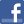Facebook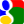Gmail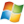Hotmail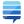StackOverflow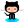GitHub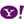Yahoo

7. Correction in function tDist? - Pavlos Stamboulides (2014-08-07 02:06)
I think I have a correction... - 0 replies
Read the whole comment and replies

6. awasome package - Maulana malik ibrahim (2013-10-13 07:30)
Read the whole comment and replies

5. Hello - Gary Bhat (2013-10-13 07:29)
Read the whole comment and replies

4. Great start - Rodney C Kite (2013-10-13 07:28)
Read the whole comment and replies

3. Nice - Nikos M. (2013-10-13 07:28)
Read the whole comment and replies

2. blog - Tom Fitzgerald (2013-10-13 07:28)Post a commentSee comments (13) Trackbacks (0)All package blogsAl-KashiAl-Kashi package blogPHP Statistics Librar...About usAdvertise on this siteSite mapNewsletterStatisticsSite tipsPrivacy policyContact
For more information send a message to `info at phpclasses dot org`.